##### The Japanese Bonsai specialist
Direct order Contact Help / Services Newsletter Pay in 3 times without charges, from 135 euros in your shopping cart (France residents only)# Cryptomeria japonica bonsai ref: 14020147

Cryptomeria japonica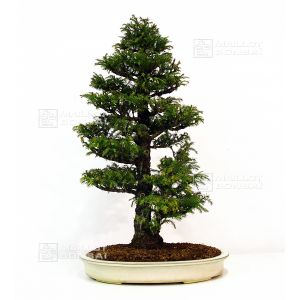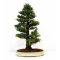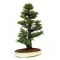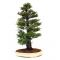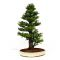ref. : 82642.360,00

voluminous and/or heavy product extra shipping of 12,00

Available quantity : 1Order

###### Description

Height of the tree only: 80 cm.

Span of the branches: 40 cm.

Measurements of the pot: 48*39*6.7 cm.

Ø of the trunk base: 8/10 cm.

Sold without shelf.

Ideal for a keen collector, this Japanese cedar (cryptomeria japonica) is sankan style with three trunks and a slim, elegant silhouette. It originates from the nursery of a famous bonsaist, Masui Mitsuo based in Kanagawa-ken prefecture. The green foliage turns rust brown in winter with the arrival of the cold weather. There are no major wounds or traces of cut branches. The main trunk has a good conical shape and established nebari at the base. Photo February 2014.

#bonsai 4.6 #cryptomeria 4.1 #japonica 3.8 #masterpieces 3.2 #branches 2.8 #trunk 2.5 #base 2.4 #with 2.4 #measurements 2.2 #established 2.1

Formule
(( ROUND((CHAR_LENGTH(b.article_nom)-CHAR_LENGTH(REPLACE(b.article_nom, 'cryptomeria', '')))/LENGTH('cryptomeria')) + ROUND((CHAR_LENGTH(b.article_description)-CHAR_LENGTH(REPLACE(b.article_description, 'cryptomeria', '')))/LENGTH('cryptomeria')) ) * 4.1) + (( ROUND((CHAR_LENGTH(b.article_nom)-CHAR_LENGTH(REPLACE(b.article_nom, 'japonica', '')))/LENGTH('japonica')) + ROUND((CHAR_LENGTH(b.article_description)-CHAR_LENGTH(REPLACE(b.article_description, 'japonica', '')))/LENGTH('japonica')) ) * 3.8) + (( ROUND((CHAR_LENGTH(b.article_nom)-CHAR_LENGTH(REPLACE(b.article_nom, 'branches', '')))/LENGTH('branches')) + ROUND((CHAR_LENGTH(b.article_description)-CHAR_LENGTH(REPLACE(b.article_description, 'branches', '')))/LENGTH('branches')) ) * 2.8) + (( ROUND((CHAR_LENGTH(b.article_nom)-CHAR_LENGTH(REPLACE(b.article_nom, 'bonsai', '')))/LENGTH('bonsai')) + ROUND((CHAR_LENGTH(b.article_description)-CHAR_LENGTH(REPLACE(b.article_description, 'bonsai', '')))/LENGTH('bonsai')) ) * 2.6) + (( ROUND((CHAR_LENGTH(b.article_nom)-CHAR_LENGTH(REPLACE(b.article_nom, 'trunk', '')))/LENGTH('trunk')) + ROUND((CHAR_LENGTH(b.article_description)-CHAR_LENGTH(REPLACE(b.article_description, 'trunk', '')))/LENGTH('trunk')) ) * 2.5) + (( ROUND((CHAR_LENGTH(b.article_nom)-CHAR_LENGTH(REPLACE(b.article_nom, 'base', '')))/LENGTH('base')) + ROUND((CHAR_LENGTH(b.article_description)-CHAR_LENGTH(REPLACE(b.article_description, 'base', '')))/LENGTH('base')) ) * 2.4) + (( ROUND((CHAR_LENGTH(b.article_nom)-CHAR_LENGTH(REPLACE(b.article_nom, 'with', '')))/LENGTH('with')) + ROUND((CHAR_LENGTH(b.article_description)-CHAR_LENGTH(REPLACE(b.article_description, 'with', '')))/LENGTH('with')) ) * 2.4) + (( ROUND((CHAR_LENGTH(b.article_nom)-CHAR_LENGTH(REPLACE(b.article_nom, 'measurements', '')))/LENGTH('measurements')) + ROUND((CHAR_LENGTH(b.article_description)-CHAR_LENGTH(REPLACE(b.article_description, 'measurements', '')))/LENGTH('measurements')) ) * 2.2) + (( ROUND((CHAR_LENGTH(b.article_nom)-CHAR_LENGTH(REPLACE(b.article_nom, 'established', '')))/LENGTH('established')) + ROUND((CHAR_LENGTH(b.article_description)-CHAR_LENGTH(REPLACE(b.article_description, 'established', '')))/LENGTH('established')) ) * 2.1) + (( ROUND((CHAR_LENGTH(b.article_nom)-CHAR_LENGTH(REPLACE(b.article_nom, 'prefecture', '')))/LENGTH('prefecture')) + ROUND((CHAR_LENGTH(b.article_description)-CHAR_LENGTH(REPLACE(b.article_description, 'prefecture', '')))/LENGTH('prefecture')) ) * 2)

## Secure payment## Delivery

Our logistic partners :